SLPS572 December   2015

PRODUCTION DATA.

1. Features
2. Applications
3. Description
4. Revision History
5. Pin Configuration and Functions
6. Specifications
7. Detailed Description
1. 7.1 Overview
2. 7.2 Functional Block Diagram
3. 7.3 Feature Description
4. 7.4 Device Functional Modes
8. Application and Implementation
1. 8.1 Application Information
2. 8.2 Typical Application
3. 8.3 System Example
9. Layout
1. 9.1 Layout Guidelines
2. 9.2 Layout Example
3. 9.3 Thermal Considerations
10. 10Device and Documentation Support
11. 11Mechanical, Packaging, and Orderable Information

• DPC|8

## 8 Application and Implementation

NOTE

Information in the following applications sections is not part of the TI component specification, and TI does not warrant its accuracy or completeness. TI’s customers are responsible for determining suitability of components for their purposes. Customers should validate and test their design implementation to confirm system functionality.

### 8.1 Application Information

The Power Stage CSD97396Q4M is a highly optimized design for synchronous buck applications using NexFET devices with a 5 V gate drive. The Control FET and Sync FET silicon are parametrically tuned to yield the lowest power loss and highest system efficiency. As a result, a rating method is used that is tailored towards a more systems centric environment. The high-performance gate driver IC integrated in the package helps minimize the parasitics and results in extremely fast switching of the power MOSFETs. System level performance curves such as Power Loss, SOA, and normalized graphs allow engineers to predict the product performance in the actual application.

### 8.2 Typical Application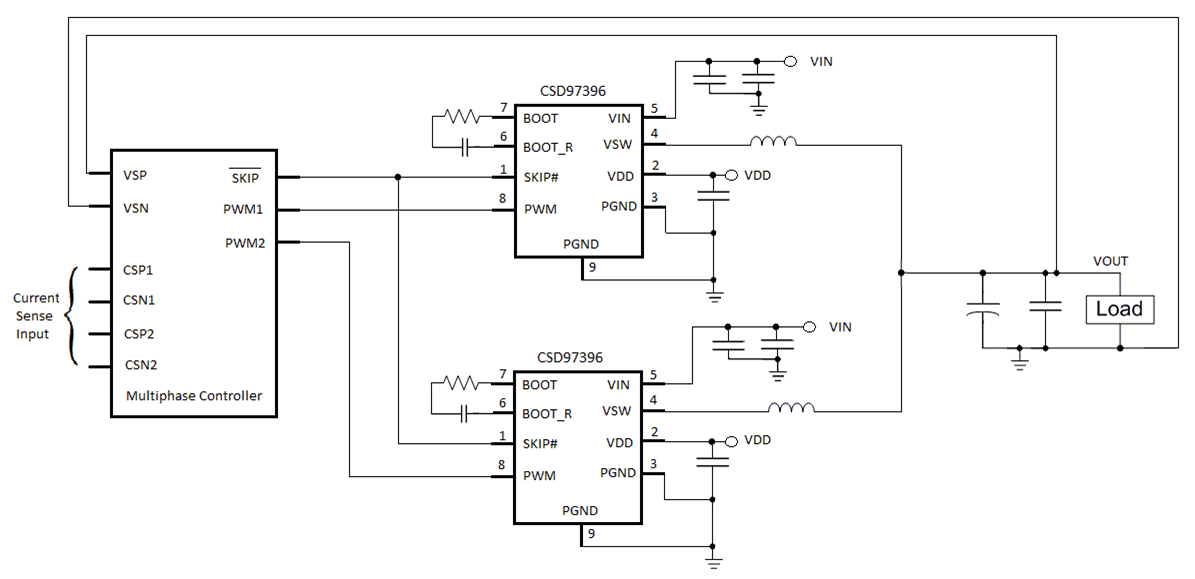Figure 3. Application Schematic

#### 8.2.1 Application Curves

TJ = 125°C, unless stated otherwise. The Typical CSD97396Q4M System Characteristic curves (see Figure 6 and Figure 7) are based on measurements made on a PCB design with dimensions of 4.0" (W) × 3.5" (L) × 0.062" (T) and 6 copper layers of 1 oz. copper thickness. See System Example for detailed explanation.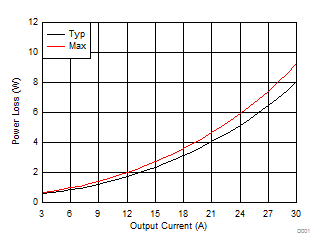VIN = 12 V VDD = 5 V VOUT = 1.8 V ƒSW = 500 kHz LOUT = 0.29 µH
Figure 4. Power Loss vs Output Current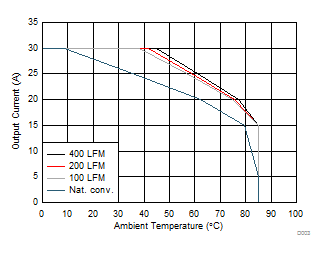VIN = 12 V VDD = 5 V VOUT = 1.8 V ƒSW = 500 kHz LOUT = 0.29 µH
Figure 6. Safe Operating Area – PCB Horizontal MountVIN = 12 V VDD = 5 V VOUT = 1.8 V IOUT = 25 A LOUT = 0.29 µH
Figure 8. Normalized Power Loss vs Frequency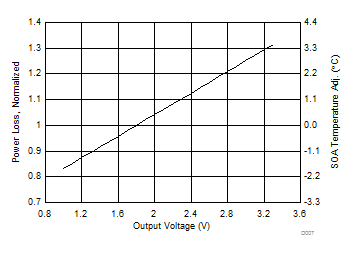VIN = 12 V VDD = 5 V IOUT = 25 A ƒSW = 500 kHz LOUT = 0.29 µH
Figure 10. Normalized Power Loss vs Output Voltage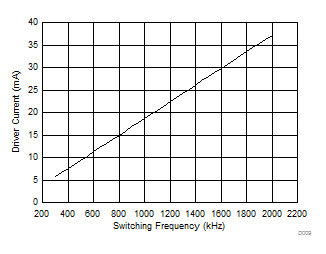VIN = 12 V VDD = 5 V IOUT = 25 A LOUT = 0.29 µH VOUT = 1.8 V
Figure 12. Driver Current vs Frequency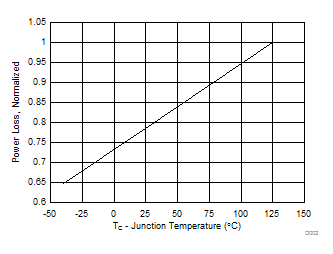VIN = 12 V VDD = 5 V VOUT = 1.8 V ƒSW = 500 kHz LOUT = 0.29 µH
Figure 5. Power Loss vs Temperature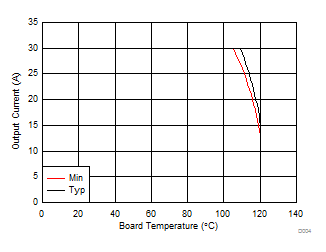VIN = 12 V VDD = 5 V VOUT = 1.8 V ƒSW = 500 kHz LOUT = 0.29 µH
Figure 7. Typical Safe Operating Area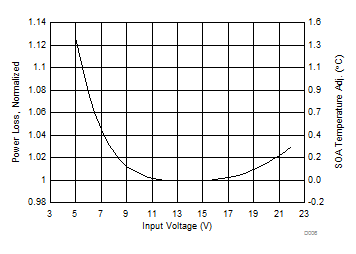IOUT = 25 A VDD = 5 V VOUT = 1.8 V ƒSW = 500 kHz LOUT = 0.29 µH
Figure 9. Normalized Power Loss vs Input Voltage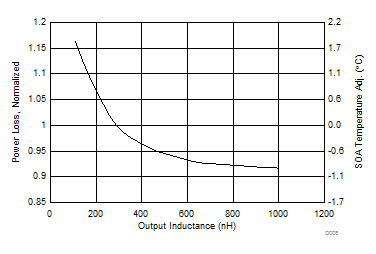VIN = 12 V VDD = 5 V IOUT = 25 A ƒSW = 500 kHz VOUT = 1.8 V
Figure 11. Normalized Power Loss vs Output InductanceVIN = 12 V VDD = 5 V VOUT = 1.8 V IOUT = 25 A LOUT = 0.29 µH
Figure 13. Driver Current vs Temperature

### 8.3 System Example

#### 8.3.1 Power Loss Curves

MOSFET centric parameters such as RDS(ON) and Qgd are primarily needed by engineers to estimate the loss generated by the devices. In an effort to simplify the design process for engineers, Texas Instruments has provided measured power loss performance curves. Figure 4 plots the power loss of the CSD97396Q4M as a function of load current. This curve is measured by configuring and running the CSD97396Q4M as it would be in the final application (see Figure 14). The measured power loss is the CSD97396Q4M device power loss which consists of both input conversion loss and gate drive loss. Equation 1 is used to generate the power loss curve.

Equation 1. Power Loss = (VIN × IIN) + (VDD × IDD) – (VSW_AVG × IOUT)

The power loss curve in Figure 4 is measured at the maximum recommended junction temperature of
TJ = 125°C under isothermal test conditions.

#### 8.3.2 SOA Curves

The SOA curves in the CSD97396Q4M datasheet give engineers guidance on the temperature boundaries within an operating system by incorporating the thermal resistance and system power loss. Figure 6 and Figure 7 outline the temperature and airflow conditions required for a given load current. The area under the curve dictates the safe operating area. All the curves are based on measurements made on a PCB design with dimensions of 4.0 inches (W) × 3.5 inches (L) × 0.062 inch (T) and 6 copper layers of 1 oz. copper thickness.

#### 8.3.3 Normalized Curves

The normalized curves in the CSD97396Q4M data sheet give engineers guidance on the Power Loss and SOA adjustments based on their application specific needs. These curves show how the power loss and SOA boundaries will adjust for a given set of systems conditions. The primary Y-axis is the normalized change in power loss and the secondary Y-axis is the change is system temperature required in order to comply with the SOA curve. The change in power loss is a multiplier for the Power Loss curve and the change in temperature is subtracted from the SOA curve.Figure 14. Power Loss Test Circuit

#### 8.3.4 Calculating Power Loss and SOA

The user can estimate product loss and SOA boundaries by arithmetic means (see the Design Example). Though the Power Loss and SOA curves in this datasheet are taken for a specific set of test conditions, the following procedure will outline the steps engineers should take to predict product performance for any set of system conditions.

#### 8.3.4.1 Design Example

Operating Conditions: Output Current (lOUT) = 20 A, Input Voltage (VIN ) = 7 V, Output Voltage (VOUT) = 2.0 V, Switching Frequency (ƒSW) = 800 kHz, Output Inductor (LOUT) = 0.2 µH

#### 8.3.4.2 Calculating Power Loss

• Typical Power Loss at 20 A = 3.71 W (Figure 4)
• Normalized Power Loss for switching frequency ≈ 1.01 (Figure 8)
• Normalized Power Loss for input voltage ≈ 1.04 (Figure 9)
• Normalized Power Loss for output voltage ≈ 1.04 (Figure 10)
• Normalized Power Loss for output inductor ≈ 1.07 (Figure 11)
• Final calculated Power Loss = 3.71 W × 1.01 × 1.04 × 1.04 × 1.07 ≈ 4.34 W

• SOA adjustment for switching frequency ≈ 0.16°C (Figure 8)
• SOA adjustment for input voltage ≈ 0.42°C (Figure 9)
• SOA adjustment for output voltage ≈ 0.46°C (Figure 10)
• SOA adjustment for output inductor ≈ 0.74°C (Figure 11)
• Final calculated SOA adjustment = 0.16 + 0.42 + 0.46 + 0.74 ≈ 1.78°C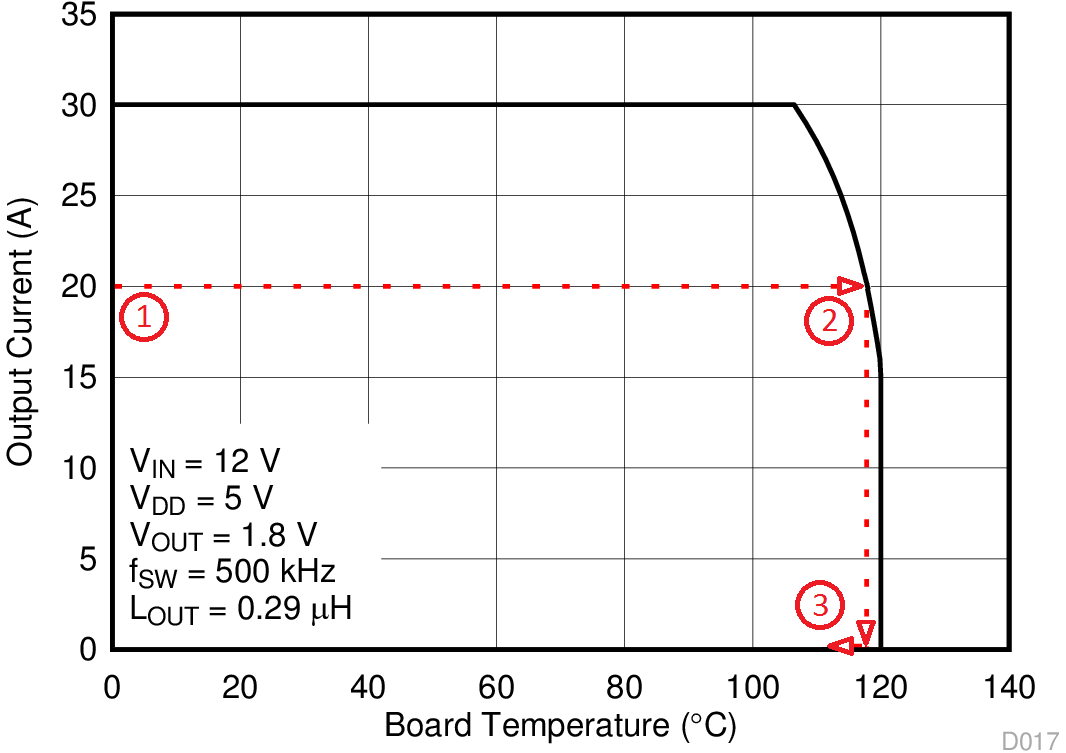Figure 15. Power Stage CSD97396Q4M SOA

In the design example above, the estimated power loss of the CSD97396Q4M would increase to 4.23 W. In addition, the maximum allowable board and/or ambient temperature would have to decrease by 1.78°C. Figure 15 graphically shows how the SOA curve would be adjusted accordingly.

1. Start by drawing a horizontal line from the application current to the SOA curve.
2. Draw a vertical line from the SOA curve intercept down to the board/ambient temperature.
3. Adjust the SOA board/ambient temperature by subtracting the temperature adjustment value.

In the design example, the SOA temperature adjustment yields a reduction in allowable board/ambient temperature of 1.78°C. In the event the adjustment value is a negative number, subtracting the negative number would yield an increase in allowable board/ambient temperature.